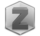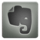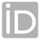You are in:Home/Publications/THE NUMERICAL SOLUTION OF LNTEGRO-PARTIAL DIFFERENTIAL EQUATIONS WITH SIXTH-DEGREE B-SPLINE FUNCTIONS

### Dr. amal foad abdelaziz soliman :: Publications:

 Title: THE NUMERICAL SOLUTION OF LNTEGRO-PARTIAL DIFFERENTIAL EQUATIONS WITH SIXTH-DEGREE B-SPLINE FUNCTIONS Authors: A. M.A. EL-ASYED; Amal F. Soliman; M. S. El-Azab Year: 2012 Keywords: Integro-partial differential equation, Time discretization, Collocation B-spline, Numerical methods. Journal: American Academic & Scholarly Research Journal Volume: 4 Issue: Not Available Pages: Not Available Publisher: Not Available Local/International: International Paper Link: Not Available Full paper amal foad abdelaziz soliman_p3.pdf Supplementary materials Not Available
 Abstract: In this paper, we consider the approximate solution of the following problem k t,s,u x,s ds f(t,x , a x b, t ( ,T) x u α x u t u t ( ( )) ) 0 2 0 2               ( , ) ( ) ( , ) ( ) (0, ) 1 2 u a t  g t , u b t  g t , t T u(x, 0)  u (x), a x  b 0 To solve this problem, we introduce a new nonstandard time discretization scheme. A proof of convergence of the approximate solution is given and error estimates are derived. The numerical results obtained by the suggested technique are compared with the exact solution of the problem. The numerical solution displays the expected convergence to the exact one as the mesh size is refined; the numerical solution displays the expected convergence to the exact one as the mesh size is refined. The numerical solution displays the expected convergence to the exact one as the mesh size is refined.